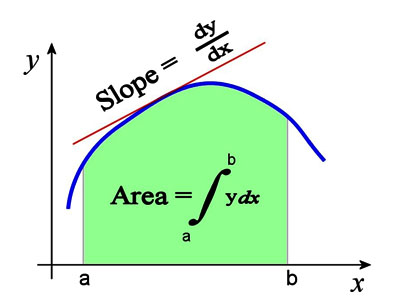ΓραμματείαSecretariat: 2410 684574 | ΦοιτητικάStudents: 2410 684387 g-ds@uth.gr
Select Page

# Mathematical Analysis

Y101

1

#### Hours/Week - ECTS

5 – 5### Learning Outcomes

This course is taught to first-year students, therefore it is a connecting link between the mathematics of the Lyceum and those that will be taught at the University. The aim of the course is to teach, in addition to rules and theorems, a mathematical way of thinking, in order to develop combinatorial and problem-solving ability.

### Indicative Module Content

• Sets. Structures. Numbers.
• Sequences and series of real numbers.
• Series of real functions.
• Differential Calculus I. Applications.
• Approximating Functions with polynomials.
• Integral Calculus I. Applications.
• Generalized (improper) integrals.
• Ordinary differential equations.
• Differential Calculus of functions of several variables (II). Applications.
• Integral Calculus of functions of several variables (II). Applications.
• Vector Calculus